# interplot: Plot the Effects of Variables in Interaction Terms

#### 2016-11-20

The interplot package is a tool for plotting the conditional coefficients (“marginal effects”) of variables included in multiplicative interaction terms. The function plots the changes in the coefficient of one variable in a two-way interaction term conditional on the value of the other included variable. The plot also includes simulated 95% confidential intervals of these coefficients. In the current version, the function works with ordinary linear regression models, generalized linear models (e.g., logit, probit, ordered logit, etc.), and multilevel (mixed-effects) regressions, all with complete or multiply imputed data.

Comparing to established alternatives such as effects::plot and sjplot::sjp.int, interplot provides a more user-friendly way to quickly produce plots that are easy to interpret. interplot plots the changes in the conditional coefficient of one variable in the interaction, rather than changes in the dependent variable itself as in the aforementioned functions. This approach avoids displaying interaction effects across multiple panels or multiple lines in favor of a single plot containing all the relevant information. Moreover, by outputting ggplot objects, interplot allows users to easily further customize their graphs.

This vignette purposes to illustrate how users can apply these functions to improve the presentation of the interactions in their models.

## Installation

To install:

• the latest released version: install.packages("interplot").
• the latest developing version: devtools::install_github("sammo3182/interplot").

## Run a Model

This example is based on the mtcars dataset, which is drawn from the 1974 volume of the US magazine Motor Trend. The dependent variable is mileage in miles per (US) gallon (mpg), and the independent variables are the number of engine cylinders (cyl) and automobile weight in thousands of pounds (wt).

data(mtcars)  #load the data

Suppose we are interested in how automobile weight affects the relationship between of the number of engine cylinders on mileage and how the number of cylinders affects the relationship between the car’s weight and its mileage. Such conditional effects are modeled using a two-way multiplicative interaction term:

m_cyl <- lm(mpg ~ wt * cyl, data = mtcars)
summary(m_cyl)
##
## Call:
## lm(formula = mpg ~ wt * cyl, data = mtcars)
##
## Residuals:
##     Min      1Q  Median      3Q     Max
## -4.2288 -1.3495 -0.5042  1.4647  5.2344
##
## Coefficients:
##             Estimate Std. Error t value Pr(>|t|)
## (Intercept)  54.3068     6.1275   8.863 1.29e-09 ***
## wt           -8.6556     2.3201  -3.731 0.000861 ***
## cyl          -3.8032     1.0050  -3.784 0.000747 ***
## wt:cyl        0.8084     0.3273   2.470 0.019882 *
## ---
## Signif. codes:  0 '***' 0.001 '**' 0.01 '*' 0.05 '.' 0.1 ' ' 1
##
## Residual standard error: 2.368 on 28 degrees of freedom
## Multiple R-squared:  0.8606, Adjusted R-squared:  0.8457
## F-statistic: 57.62 on 3 and 28 DF,  p-value: 4.231e-12

The coefficient of the interactive term (wt:cyl) is positive and statistically significant at .05 level. This tells us that the coefficient of wt depends on the value of cyl and vice versa; these estimated coefficients are conditional. It does not indicate anything, however, about the magnitude or statistical significance of these conditional coefficients. A plot produced by interplot easily and clearly answers these latter questions.

## Create a Basic Graph of the Conditional Coefficients

To plot conditional coefficients, a user needs to provide only three basic pieces of information: the object of a regression result (m), the variable whose coefficient is to be plotted (var1), and the variable on which the coefficient is conditional (var2). Taking the previous example, if we intend to know how the weight of a car can affect the coefficient for number of cylinders on the mileage, var1 is cyl, and var2 is wt.

library(interplot)

interplot(m = m_cyl, var1 = "cyl", var2 = "wt")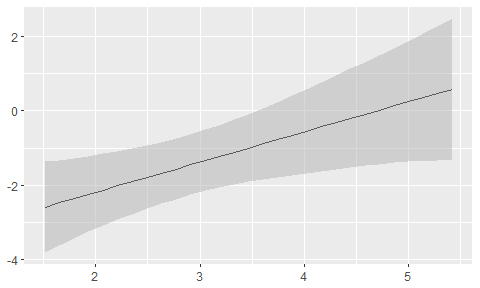The plot clearly shows that with increasing automobile weight (along the x axis), the magnitude of the coefficient of the number of cylinders on the mileage also increases (along the y axis).

Similarly, to show how the number of cylinders affects the coefficient of automobile weight on mileage, one only needs to switch var1 and var2:

interplot(m = m_cyl, var1 = "wt", var2 = "cyl")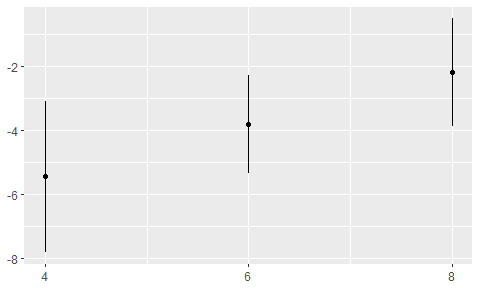Users can adjust the CI level by setting the ci option. The default value is 95% CIs. The following example resets the the CIs to 90%.

interplot(m = m_cyl, var1 = "wt", var2 = "cyl", ci = .9, point = T)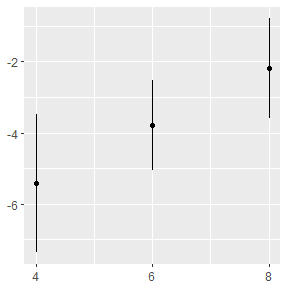The format of the plot also changes when wt is var1 and cyl is var2. This is because cyl is not a continuous variable but a categorical one with just three values: 4, 6, and 8. interplot automatically detects the number of values taken on by var2 and chooses the appropriate plot format. If there are fewer than 10 values, the function will produce a “dot-and-whisker” plot; otherwise, by default, it will generate a “line-and-ribbon” plot. Users may override this default by setting the argument point to TRUE.

interplot(m = m_cyl, var1 = "cyl", var2 = "wt", point = T) +
# changing the angle of x labels for a clearer vision
theme(axis.text.x  = element_text(angle=90))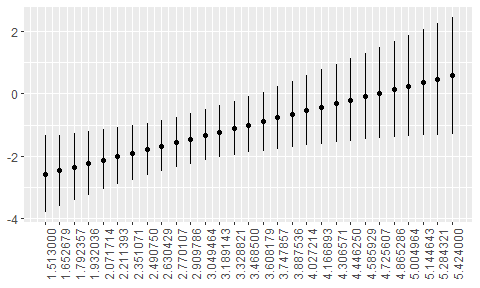## Change the Appearance of the Plot

Plots generated by interplot are, by design, very basic. They are, however, ggplot objects and so may be easily modified further.

interplot(m = m_cyl, var1 = "cyl", var2 = "wt") +
# Add labels for X and Y axes
xlab("Automobile Weight (thousands lbs)") +
ylab("Estimated Coefficient for\nNumber of Cylinders") +
# Change the background
theme_bw() +
ggtitle("Estimated Coefficient of Engine Cylinders \non Mileage by Automobile Weight") +
theme(plot.title = element_text(face="bold")) +
# Add a horizontal line at y = 0
geom_hline(yintercept = 0, linetype = "dashed")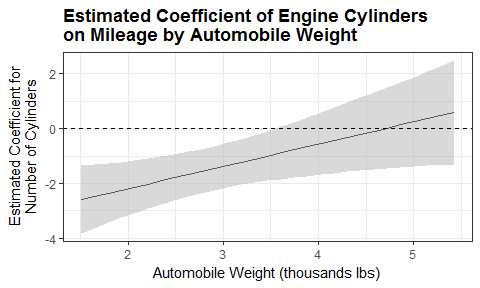For the default settings of the whisker or ribbon, the users can also use arguments, such as ercolor and esize to modify. More arguments can be found in the ?interplot file.

interplot(m = m_cyl, var1 = "wt", var2 = "cyl", ercolor = "blue", esize = 1.5) +
geom_point(size = 2, color = "red")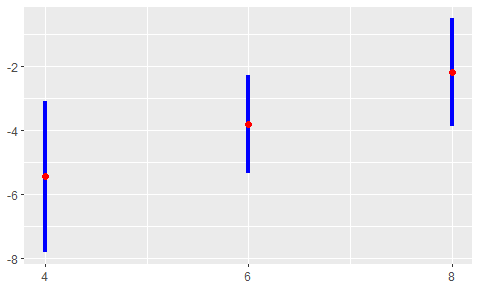## Plot the Effect of a Variable with a Quadratic Term

The simplest type of interaction is quadratic term, which can be regarded as a variable interact with itself. interplot can visualize this case when the variable names of var1 and var2 are the same.

m_wt <- lm(mpg ~ wt + I(wt^2), data = mtcars)

interplot(m = m_wt, var1 = "wt", var2 = "wt")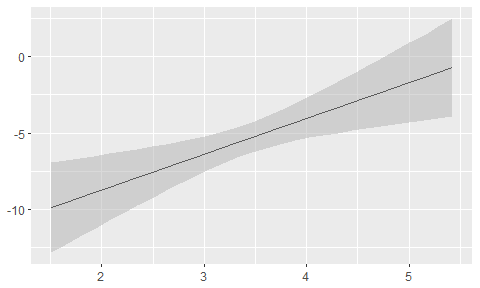## Plot Interactions with a Factor Term

When either the conditioned or the conditioning base term of an interaction is a factor, interplot creates a facet in which the conditional effect under each category of the factor is visualized in a separate panel.

mtcars$gear <- factor(mtcars$gear)
m_gear <- lm(mpg ~ gear * wt, data = mtcars)

interplot(m = m_gear, var1 = "wt", var2 = "gear")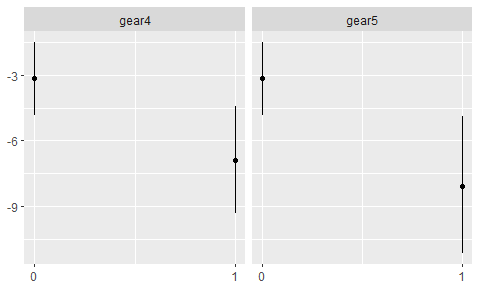## Include the Distribution of the Conditioning Variable

Berry, Golder, and Milton (2012) points out that, when a variable’s conditional effect reaches statistical significance over only part of the range of the conditioning variable, it can be helpful to the evaluation of the substantive significance of the conditional effect to know the distribution of the conditioning variable. For this purpose, interplot has the hist argument for users to choose to superimpose a histogram at the bottom of the conditional effect plot.

interplot(m = m_cyl, var1 = "cyl", var2 = "wt", hist = TRUE) +
geom_hline(yintercept = 0, linetype = "dashed")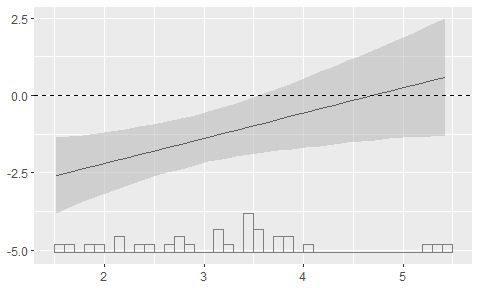Our implementation of this option was inspired by the excellent work of Hainmueller, Mummolo, and Xu (2016). A tip is that when presenting the histogram, some default setting would not be directly modified by the build-in arguments or the geom functions. Instead, one can change these settings by the aes function—as illustrated by the following example.

interplot(m = m_cyl, var1 = "cyl", var2 = "wt", hist = TRUE) +
aes(color = "pink") + theme(legend.position="none") +  # geom_line(color = "pink") +
geom_hline(yintercept = 0, linetype = "dashed")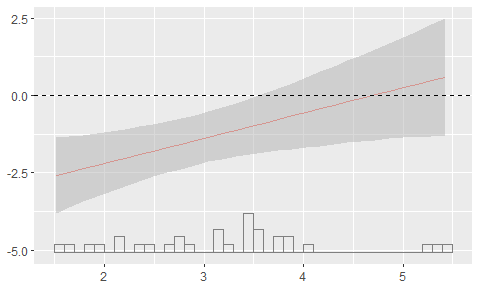## Advanced Use: Plot Conditional Effects Without a Model

In some cases, one may analyze some complicated or self-made regression functions which are not supported by the current version of interplot. For such models, as long as the user has a dataset loading the simulated results of the interaction effects, she can still use interplot to visualize it. The dataset needs four columns the scale of the conditioning variable (fake), the simulated interactive effect at each break of the conditioning variable (coef1), and the simulated lower bound and upper bound of the confidence interval (lb, and ub). The column names should be exactly the ones shown in the above parentheses. Here is an example with some arbitrary artificial data:

# Create a fake dataset of conditional effects
fake <- rnorm(100, 0, 1)
coef1 <- fake * sample(.5:2.5, 100, replace = T)
lb <- coef1 - .5
ub <- coef1 + .5

df_fake <- data.frame(cbind(fake, coef1, lb, ub))

# Use interplot directly with the dataset
interplot(df_fake)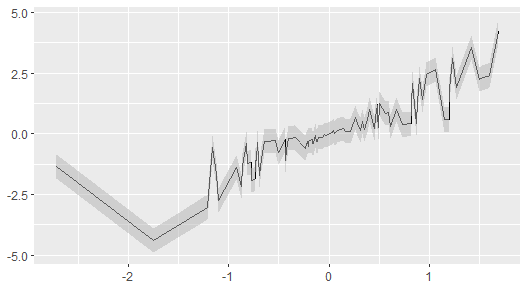If one also has the data of the var2, she can also draw a histogram under it by the argument var_dt.

var2_fake <- fake
# Set hist to TRUE is required to superimpose a histogram.
interplot(df_fake, hist = TRUE, var2_dt = var2_fake)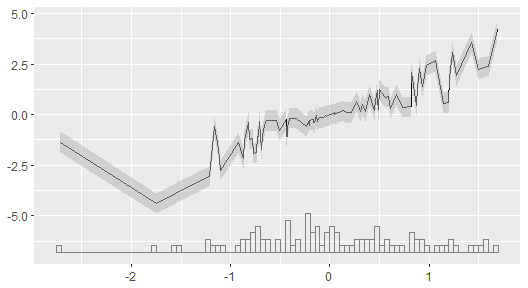## The ribbon and histogram do not fit. This is just an illustration

## Conclusion

The interplot package provides a flexible and convenient way to visualize conditional coefficients of variables in multiplicative interaction terms. This vignette offers an overview of its use and features. We encourage users to consult the help files for more details.

## Affiliation

Frederick Solt

Department of Political Science,

University of Iowa,

324 Schaeffer Hall,

20 E Washington St, Iowa City, IA, 52242

Email: frederick-solt@uiowa.edu

Website: http://myweb.uiowa.edu/fsolt

Yue Hu

Department of Political Science,

University of Iowa,

313 Schaeffer Hall,

20 E Washington St, Iowa City, IA, 52242

Email: yue-hu-1@uiowa.edu

## Reference

Berry, William D, Matt Golder, and Daniel Milton. 2012. “Improving Tests of Theories Positing Interaction.” Journal of Politics 74 (3). Cambridge Univ Press: 653–71.

Hainmueller, Jens, Jonathan Mummolo, and Yiqing Xu. 2016. “How Much Should We Trust Estimates from Multiplicative Interaction Models? Simple Tools to Improve Empirical Practice.”